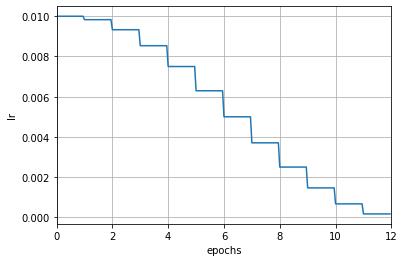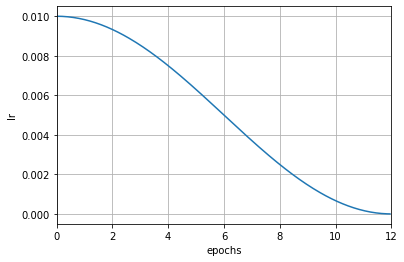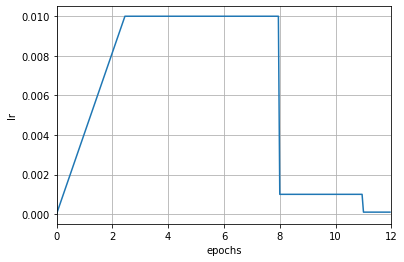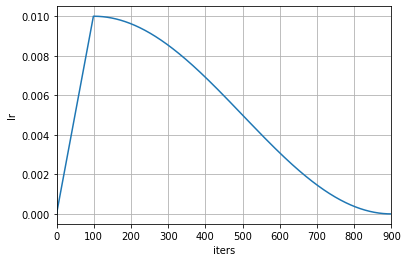Shortcuts

# 优化器参数调整策略（Parameter Scheduler）¶

## 参数调度器的使用¶

import torch
from torch.optim import SGD
from torch.optim.lr_scheduler import ExponentialLR

model = torch.nn.Linear(1, 1)
dataset = [torch.randn((1, 1, 1)) for _ in range(20)]
optimizer = SGD(model, 0.1)
scheduler = ExponentialLR(optimizer, gamma=0.9)

for epoch in range(10):
for data in dataset:
output = model(data)
loss = 1 - output
loss.backward()
optimizer.step()
scheduler.step()


mmengine.optim.scheduler 中，我们支持大部分 PyTorch 中的学习率调度器，例如 ExponentialLRLinearLRStepLRMultiStepLR 等，使用方式也基本一致，所有支持的调度器见调度器接口文档。同时增加了对动量的调整，在类名中将 LR 替换成 Momentum 即可，例如 ExponentialMomentumLinearMomentum。更进一步地，我们实现了通用的参数调度器 ParamScheduler，用于调整优化器的中的其他参数，包括 weight_decay 等。这个特性可以很方便地配置一些新算法中复杂的调整策略。

### 使用单一的学习率调度器¶

# 基于手动构建学习率调度器的例子
from torch.optim import SGD
from mmengine.runner import Runner
from mmengine.optim.scheduler import MultiStepLR

optimizer = SGD(model.parameters(), lr=0.01, momentum=0.9)
param_scheduler = MultiStepLR(optimizer, milestones=[8, 11], gamma=0.1)

runner = Runner(
model=model,
optim_wrapper=dict(
optimizer=optimizer),
param_scheduler=param_scheduler,
...
)# 在配置文件中设置学习率调度器字段
param_scheduler = dict(type='MultiStepLR', by_epoch=True, milestones=[8, 11], gamma=0.1)


param_scheduler = dict(type='MultiStepLR', by_epoch=False, milestones=[600, 800], gamma=0.1)epoch_length = len(train_dataloader)
param_scheduler = MultiStepLR.build_iter_from_epoch(optimizer, milestones=[8, 11], gamma=0.1, epoch_length=epoch_length)


param_scheduler = dict(type='MultiStepLR', by_epoch=True, milestones=[8, 11], gamma=0.1, convert_to_iter_based=True)


param_scheduler = dict(type='CosineAnnealingLR', by_epoch=True, T_max=12)param_scheduler = dict(type='CosineAnnealingLR', by_epoch=True, T_max=12, convert_to_iter_based=True)### 组合多个学习率调度器（以学习率预热为例）¶

MMEngine 支持组合多个调度器一起使用，只需将配置文件中的 scheduler 字段修改为一组调度器配置的列表，SchedulerStepHook 可以自动对调度器列表进行处理。下面的例子便实现了学习率预热。

param_scheduler = [
# 线性学习率预热调度器
dict(type='LinearLR',
start_factor=0.001,
by_epoch=False,  # 按迭代更新学习率
begin=0,
end=50),  # 预热前 50 次迭代
# 主学习率调度器
dict(type='MultiStepLR',
by_epoch=True,  # 按轮次更新学习率
milestones=[8, 11],
gamma=0.1)
]param_scheduler = [
# 在 [0, 100) 迭代时使用线性学习率
dict(type='LinearLR',
start_factor=0.001,
by_epoch=False,
begin=0,
end=100),
# 在 [100, 900) 迭代时使用余弦学习率
dict(type='CosineAnnealingLR',
T_max=800,
by_epoch=False,
begin=100,
end=900)
]## 如何调整其他参数¶

### 动量¶

param_scheduler = [
# the lr scheduler
dict(type='LinearLR', ...),
# 动量调度器
dict(type='LinearMomentum',
start_factor=0.001,
by_epoch=False,
begin=0,
end=1000)
]


### 通用的参数调度器¶

MMEngine 还提供了一组通用的参数调度器用于调度优化器的 param_groups 中的其他参数，将学习率调度器类名中的 LR 改为 Param 即可，例如 LinearParamScheduler。用户可以通过设置参数调度器的 param_name 变量来选择想要调度的参数。

param_scheduler = [
dict(type='LinearParamScheduler',
param_name='lr',  # 调度 optimizer.param_groups 中名为 'lr' 的变量
start_factor=0.001,
by_epoch=False,
begin=0,
end=1000)
]


param_scheduler = [
dict(type='LinearParamScheduler',
param_name='weight_decay',  # 调度 optimizer.param_groups 中名为 'weight_decay' 的变量
start_factor=0.001,
by_epoch=False,
begin=0,
end=1000)
]


© Copyright 2022, mmengine contributors. Revision eb2dc671.

Built with Sphinx using a theme provided by Read the Docs.
Versions
latest
stable
v0.7.4
v0.7.3
v0.7.2
v0.7.1
v0.7.0
v0.6.0
v0.5.0
v0.4.0
v0.3.0
v0.2.0